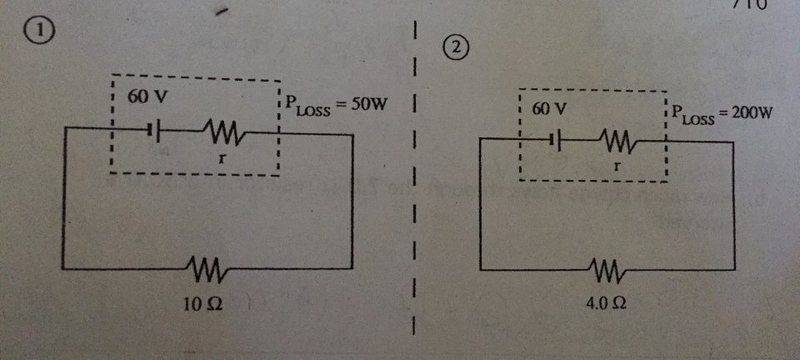# Find Internal Resistance From 2 Circuits w/ The Same Battery

Aleena753

## Homework Statement

A battery with an emf of 60 V and an internal resistance (r) is connected to a 10 ohm external resistance. The power lost inside the battery is 50w. The same battery is then connected to a 4.0 ohm resistance. The power loss is 200w. What is the internal resistance of the battery?V = emf - Irint

P= VI

V= IR

P=I^2R

P= V^2/R

## The Attempt at a Solution

P= V^2/R
V= √ PR
V= √50 x 10
V= 22.36 V

I= V/R
= 22.36/ 10
= 2.23 A
Rint= emf - V/ I
Rint= 60 - 22.3/ 2.23
= 16.9 ohms

This is obviously wrong and I didn't even incorporate the second circuit yet. I'm pretty sure I could finish this question if I knew how to start. Any help is appreciated :)

Last edited:

Staff Emeritus